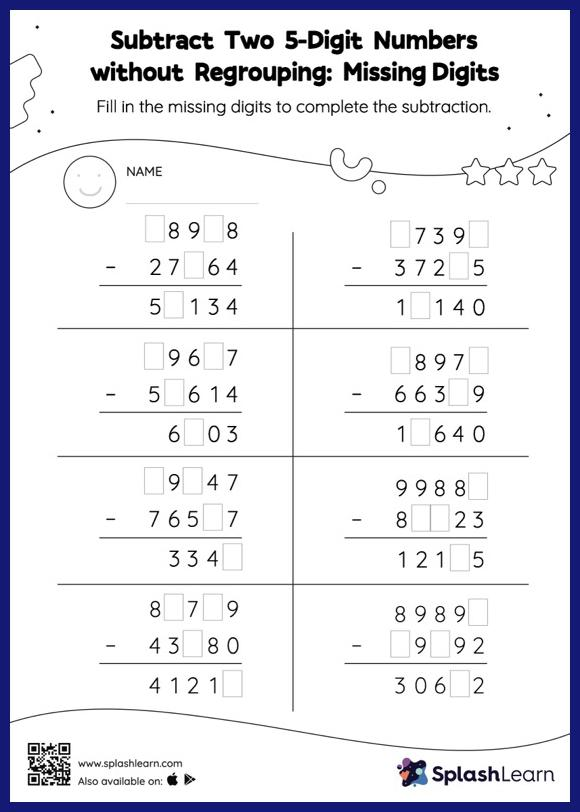# Subtract Two 5-Digit Numbers without Regrouping: Missing Digits Worksheet

Home > Subtract Two 5-Digit Numbers without Regrouping: Missing DigitsThis subtract two 5-digit numbers without regrouping worksheet consists of tasks to help your young mathematician develop fluency with subtraction. Students use the count back strategy or the relationship between addition and subtraction to find the missing number in this worksheet. However, they do not need to regroup numbers to get to the answer in subtract two 5-digit numbers without regrouping worksheet. This worksheet is about practicing the column method in which numbers are written one on top of another. In this method, students use their place value understanding to solve the problems.